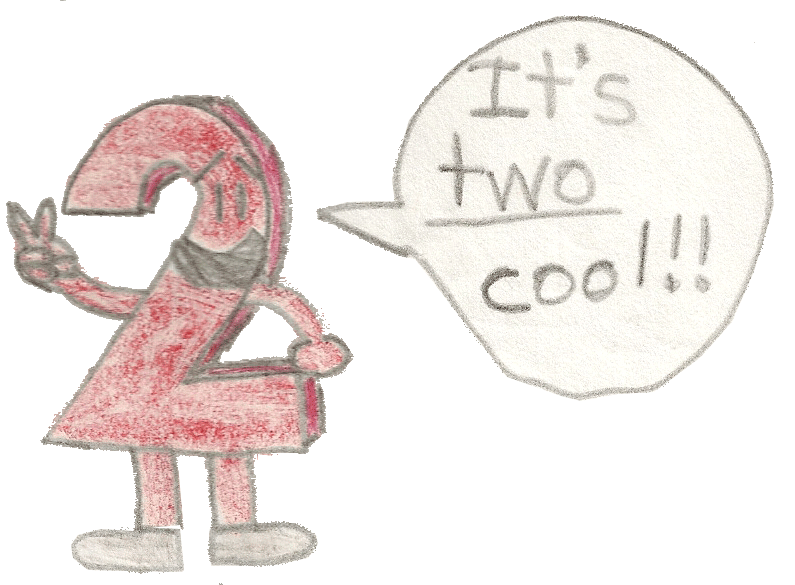# Perimeter Punch!

## Example:

Suppose the 3 sides of a triangle are:

Side A: 3 units

Side B: 4 units

Side C: 5 units

Perimeter of triangle = 12 units

Area of triangle = A * B * ½ = 6 square units

(By the way, A is the height & B is the base!)

Now watch what happens when the length of each side is multiplied by the square root of 2:

Side A: 3√(2) units

Side B: 4√(2) units

Side C: 5√(2) units

Perimeter of triangle = 12√(2) units

Area of triangle = A * B * ½ = 12 square units

(And 6 * 2 = 12)

## What happened?

The square root of 2 got squared during the multiplication in the Area formula. Obviously, squaring the square root gives you the original number under the radical sign, which in this case is 2; so the area gets doubled! (In other words, multiplied by 2) However, ½ is the reciprocal of 2, so their product is 1, which is the number of multiplicative identity. The number ½ is a constant in the formula for the area of a triangle since a triangle is half of a rectangle. Cool, huh?Another thing: All of the angles of the polygon stayed exactly the same. If you resize at least 1 side without resizing the other sides, then that'll cause the angles to change! But you can resize all of the sides simultaneously & every angle will stay exactly the same! This is called congruency.